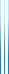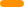# PublicationsPapers

## Space complexity in polynomial calculus

Yuval Filmus, Massimo Lauria, Jakob Nordström, Neil Thapen and Noga Zewi
CCC 2012, SICOMP

During the last decade, an active line of research in proof complexity has been to study space complexity and time-space trade-offs for proofs. Besides being a natural complexity measure of intrinsic interest, space is also an important issue in SAT solving, and so research has mostly focused on weak systems that are used by SAT solvers.

There has been a relatively long sequence of papers on space in resolution, which is now reasonably well understood from this point of view. For other natural candidates to study, however, such as polynomial calculus or cutting planes, very little has been known. We are not aware of any nontrivial space lower bounds for cutting planes, and for polynomial calculus the only lower bound has been for CNF formulas of unbounded width in Alekhnovich et al., where the space lower bound is smaller than the initial width of the clauses in the formulas. Thus, in particular, it has been consistent with current knowledge that polynomial calculus could be able to refute any $k$-CNF formula in constant space.

We prove several new results on space in polynomial calculus (PC), and in the extended proof system polynomial calculus resolution (PCR) studied by Alekhnovich et al.:

• We prove an $\Omega(n)$ space lower bound in PC for the canonical 3-CNF version of the pigeonhole principle formulas with $m$ pigeons and $n$ holes, and show that this is tight.
• For PCR, we prove an $\Omega(n)$ space lower bound for a bitwise encoding of the functional pigeonhole principle. These formulas have width $O(\log n)$, and hence this is an exponential improvement over Alekhnovich et al. measured in the width of the formulas.
• We then present another encoding of the pigeonhole principle that has constant width, and prove an $\Omega(n)$ space lower bound in PCR for these formulas as well.
• Finally, we prove that any $k$-CNF formula can be refuted in PC in simultaneous exponential size and linear space (which holds for resolution and thus for PCR, but was not obviously the case for PC). We also characterize a natural class of CNF formulas for which the space complexity in resolution and PCR does not change when the formula is transformed into 3-CNF in the canonical way, something that we believe can be useful when proving PCR space lower bounds for other well-studied formula families in proof complexity.

## BibTeX

@inproceedings{FLNTZ2012,
author = {Yuval Filmus and Massimo Lauria and Jakob Nordstr\"om and
Neil Thapen and Noga Zewi},
title = {Space complexity in polynomial calculus},
booktitle = {The 27th Annual Conference on Computational Complexity ({CCC} 2012)},
year = {2012}
}
@article{FLNTZ2015,
author = {Yuval Filmus and Massimo Lauria and Jakob Nordstr\"om and
Neil Thapen and Noga Zewi},
title = {Space complexity in polynomial calculus},
journal = {SIAM J. Comput.},
volume = {44},
number = {4},
pages = {1119--1153},
year = {2015}
}copy to clipboard